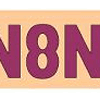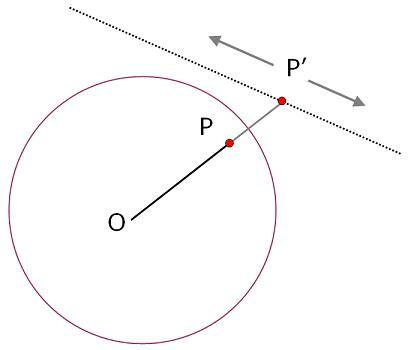#### You may also like### Symmetric Trace

Points off a rolling wheel make traces. What makes those traces have symmetry?### Mapping the Wandering Circle

In the diagram the point P can move to different places around the dotted circle. Each position P takes will fix a corresponding position for P'. As P moves around on that circle what will P' do?### Like a Circle in a Spiral

A cheap and simple toy with lots of mathematics. Can you interpret the images that are produced? Can you predict the pattern that will be produced using different wheels?

# The Line and Its Strange Pair

##### Age 14 to 16 Challenge Level:This problem builds on from Points in Pairs - you may wish to look at that first.

Two points, one inside a circle and the other outside, are related in the following way :

A line starting at the centre of the circle and passing through the first point ( P ) goes on to pass through the second point ( P' ).

Positions along the line are such that the ratio of OP to the radius of the circle matches the ratio of the radius of the circle to OP'.

For example if OP happened to be 2/3 of the radius then OP' would be 3/2 of the radius.

In the diagram above, the point P' can move to different places along the dotted line.

Each position P' takes will fix a corresponding position for P.

If P' moves along a straight line what does P do?

Why?

You might find it useful to use the interactivity below. Holding down the mouse button will leave a trail.

Full Screen Version